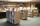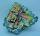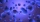Logarithm + geometric progression - examples

1. CrystalCrystal grows every month 1.9 promile of its mass. For how many months to grow a crystal from weight 136 g to 384 g?
2. Compound interestCalculate time when deposit in the bank with interest 2.5% p.a. doubles.
3. DemographicsThe population grew in the city in 10 years from 42000 to 54500. What is the average annual percentage increase of population?
4. Geometric sequence 3In geometric sequence is a8 = 312500; a11= 39062500; sn=1953124. Calculate the first item a1, quotient q and n - number of members by their sum s_n.
5. SequenceCalculate what member of the sequence specified by ? has value 86.
6. PopulationThe town has 65,000 inhabitants. 40 years ago there were 157,000. How many people will live in town in 10 years if the average rate in population is as in previous years?
7. Future of librariesYou know that thanks to the Internet, electronic communications and developing online resources on the Internet annually declining number of traditional readership by 17%. These deal is irreversible evolutionary shift from old books from libraries to immed
8. Half lifeDetermine the half life of bismuth, when bismuth weight from the original weight of 32 g was only 2 grams in 242 minutes.
9. To the cinemaJane at 8 am got message that all 1093 school pupils will go to the cinema. Within 20 min she said it to the three friends. Each of them again for 20 minutes said to the other three. In this way the message spread further. At what time all the children in.
10. Geometric sequenceIn the geometric sequence is a4 = 20 a9 = -160. Calculate the first member a1 and quotient q.
11. Geometric progressionIn geometric progression, a1 = 7, q = 5. Find the condition for n to sum first n members is: sn≤217.
12. MicroorganismsThe first generation of micro-organisms has a population of 13500 members. Each next generation is 11/10 times the previous one. Find out how many generations will reach at least three times members of the first generation.

We apologize, but in this category are not a lot of examples.
Do you have an interesting mathematical example that you can't solve it? Enter it, and we can try to solve it.

To this e-mail address, we will reply solution; solved examples are also published here. Please enter e-mail correctly and check whether you don't have a full mailbox.